# Types of Graphs and Charts And Their Uses

If you are wondering what are the different types of graphs and charts, their uses and names, this page summarizes them with examples and pictures.

As the different kinds of graphs aim to represent data, they are used in many areas such as: in statistics, in data science, in math, in economics, in business and etc.

Every type of graph is a visual representation of data on diagram plots (ex. bar, pie, line chart) that show different types of graph trends and relationships between variables.

Although it is hard to tell what are all the types of graphs, this page consists all of the common types of statistical graphs and charts (and their meanings) widely used in any science.

1. Line Graphs

A line chart graphically displays data that changes continuously over time. Each line graph consists of points that connect data to show a trend (continuous change). Line graphs have an x-axis and a y-axis. In the most cases, time is distributed on the horizontal axis.

Uses of line graphs:

• When you want to show trends. For example, how house prices have increased over time.
• When you want to make predictions based on a data history over time.
• When comparing two or more different variables, situations, and information over a given period of time.

Example:

The following line graph shows annual sales of a particular business company for the period of six consecutive years: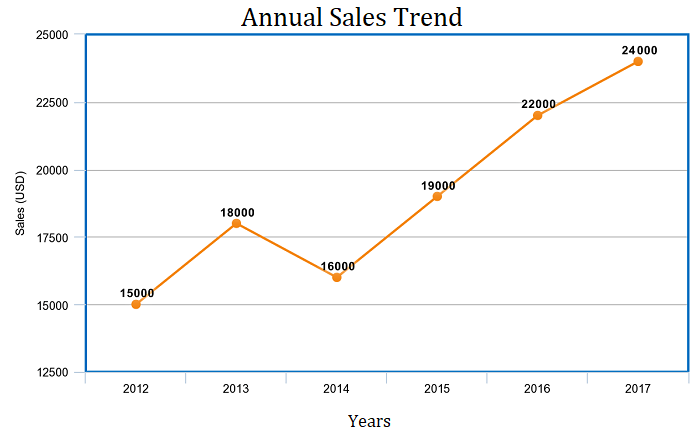Note: the above example is with 1 line. However, one line chart can compare multiple trends by several distributing lines.

2. Bar Charts

Bar charts represent categorical data with rectangular bars (to understand what is categorical data see categorical data examples). Bar graphs are among the most popular types of graphs and charts in economics, statistics, and marketing. They are commonly used to compare several categories of data.

Each rectangular bar has length and height proportional to the values that they represent.

One axis of the bar chart presents the categories being compared. The other axis shows a measured value.

Bar Charts Uses:

• When you want to display data that are grouped into nominal or ordinal categories (see nominal vs ordinal data).
• To compare data among different categories.
• Bar charts can also show large data changes over time.
• Bar charts are ideal for visualizing the distribution of data when we have more than three categories.

Example:

The bar chart below represents the total sum of sales for Product A and Product B over three years.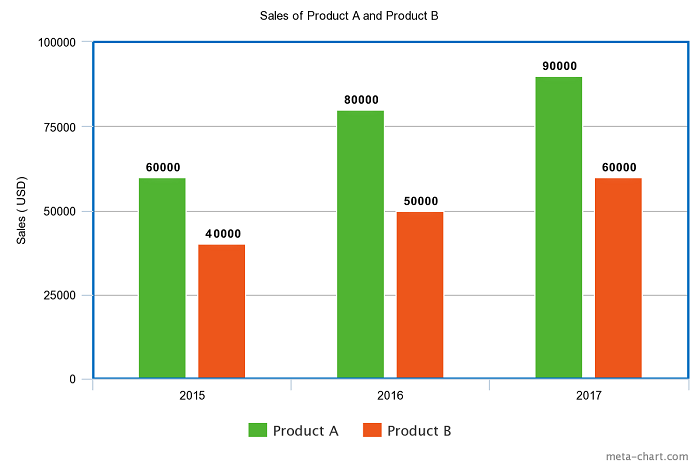The bars are 2 types: vertical or horizontal. It doesn’t matter which kind you will use. The above one is a vertical type.

3. Pie Charts

When it comes to statistical types of graphs and charts, the pie chart (or the circle chart) has a crucial place and meaning. It displays data and statistics in an easy-to-understand ‘pie-slice’ format and illustrates numerical proportion.

Each pie slice is relative to the size of a particular category in a given group as a whole. To say it in another way, the pie chart brakes down a group into smaller pieces. It shows part-whole relationships.

To make a pie chart, you need a list of categorical variables and numerical variables.

Pie Chart Uses:

• When you want to create and represent the composition of something.
• It is very useful for displaying nominal or ordinal categories of data.
• To show percentage or proportional data.
• When comparing areas of growth within a business such as profit.
• Pie charts work best for displaying data for 3 to 7 categories.

Example:

The pie chart below represents the proportion of types of transportation used by 1000 students to go to their school.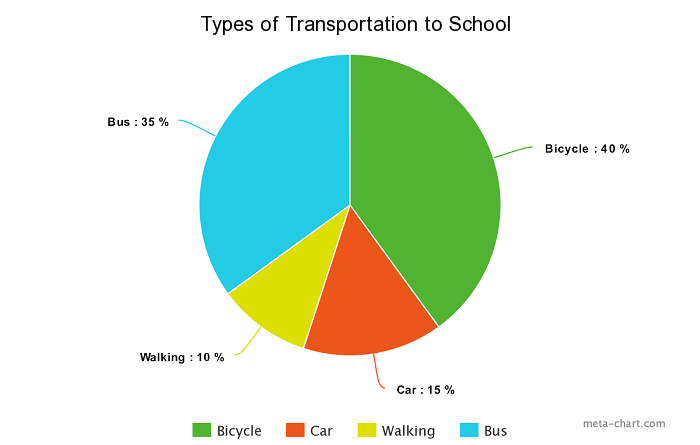Pie charts are widely used for displaying marketing data.

4. Histogram

A histogram shows continuous data in ordered rectangular columns (to understand what is continuous data see our post discrete vs continuous data). Usually, there are no gaps between the columns.

The histogram displays a frequency distribution (shape) of a data set. At first glance, histograms look alike to bar graphs. However, there is a key difference between them. Bar Chart represents categorical data and histogram represent continuous data.

Histogram Uses:

• When the data is continuous.
• When you want to represent the shape of the data’s distribution.
• When you want to see whether the outputs of two or more processes are different.
• To summarize large data sets graphically.
• To communicate the data distribution quickly to others.

Example:

The histogram below represents per capita income for five age groups.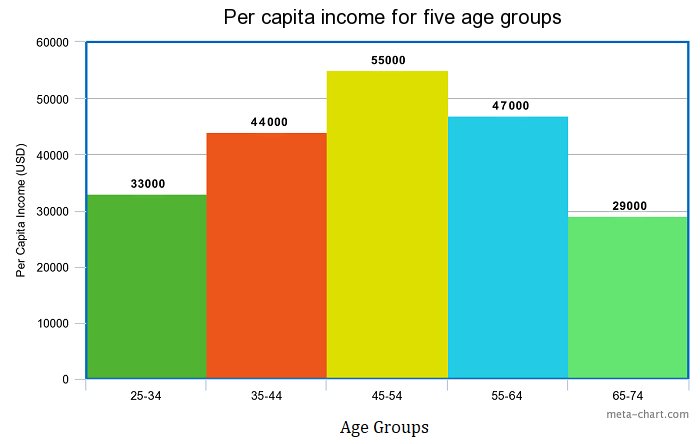Histograms are very widely used in statistics, business, and economics.

5. Scatter plot

The scatter plot is an X-Y diagram that shows a relationship between two variables. It is used to plot data points on a vertical and a horizontal axis. The purpose is to show how much one variable affects another.

Usually, when there is a relationship between 2 variables, the first one is called independent. The second variable is called dependent because its values depend on the first variable.

Scatter plots also help you predict the behavior of one variable (dependent) based on the measure of the other variable (independent).

Scatter plot uses:

• When trying to find out whether there is a relationship between 2 variables.
• To predict the behavior of dependent variable based on the measure of the independent variable.
• When having paired numerical data.
• When working with root cause analysis tools to identify the potential for problems.
• When you just want to visualize the correlation between 2 large datasets without regard to time.

Example:

The below Scatter plot presents data for 7 online stores, their monthly e-commerce sales, and online advertising costs for the last year.The orange line you see in the plot is called “line of best fit” or a “trend line”. This line is used to help us make predictions that are based on past data.

The Scatter plots are used widely in data science and statistics. They are a great tool for visualizing linear regression models.

More examples and explanation for scatter plots you can see in our post what does a scatter plot show and simple linear regression examples.

6. Venn Chart

Venn Diagram (also called primary diagram, set diagram or logic diagrams) uses overlapping circles to visualize the logical relationships between two or more group of items.

Venn Diagram is one of the types of graphs and charts used in scientific and engineering presentations, in computer applications, in maths, and in statistics.

The basic structure of the Venn diagram is usually overlapping circles. The items in the overlapping section have specific common characteristics. Items in the outer portions of the circles do not have common traits.

Venn Chart Uses:

• When you want to compare and contrast groups of things.
• To categorize or group items.
• To illustrate logical relationships from various datasets.
• To identify all the possible relationships between collections of datasets.

Example:

The following science example of Venn diagram compares the features of birds and bats.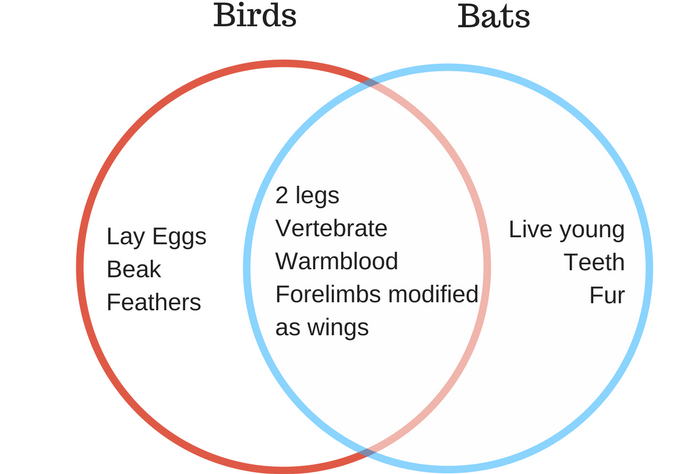7. Area Charts

Area charts show the change in one or several quantities over time. They are very similar to the line chart. However, the area between axis and line are usually filled with colors.

Despite line and area charts support the same type of analysis, they cannot be always used interchangeably. Line charts are often used to represent multiple data sets. Area charts cannot show multiple data sets clearly because area charts show a filled area below the line.

Area Chart Uses:

• When you want to show trends, rather than express specific values.
• To show a simple comparison of the trend of data sets over the period of time.
• To display the magnitude of a change.
• To compare a small number of categories.

The area chart has 2 variants: a variant with data plots overlapping each other and a variant with data plots stacked on top of each other (known as stacked area chart – as the shown in the following example).

Example:

The area chart below shows quarterly sales for product categories A and B for the last year.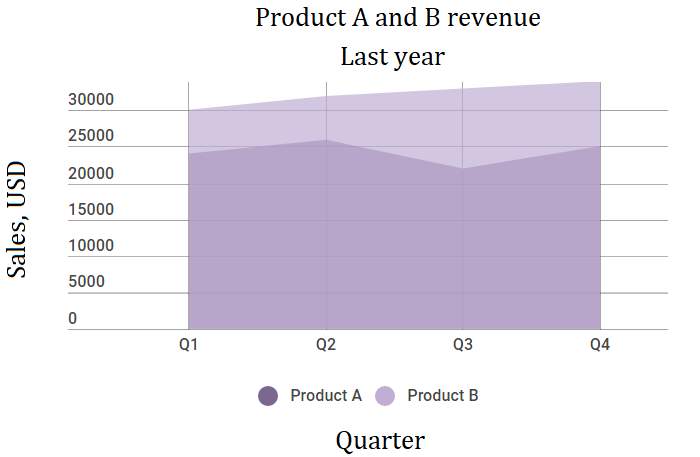This area chart shows you a quick comparison of the trend in the quarterly sales of Product A and Product B over the period of the last year.

8. Spline Chart

The Spline Chart is one of the most widespread types of graphs and charts used in statistics. It is a form of the line chart that represent smooth curves through the different data points.

Spline charts possess all the characteristics of a line chart except that spline charts have a fitted curved line to join the data points. In comparison, line charts connect data points with straight lines.

Spline Chart Uses:

• When you want to plot data that requires the usage of curve-fitting such as a product lifecycle chart or an impulse-response chart.
• Spline charts are often used in designing Pareto charts.
• Spline chart also is often used for data modeling by when you have limited number of data points and estimating the intervening values.

Example:

The following spline chart example shows sales of a company through several months of a year: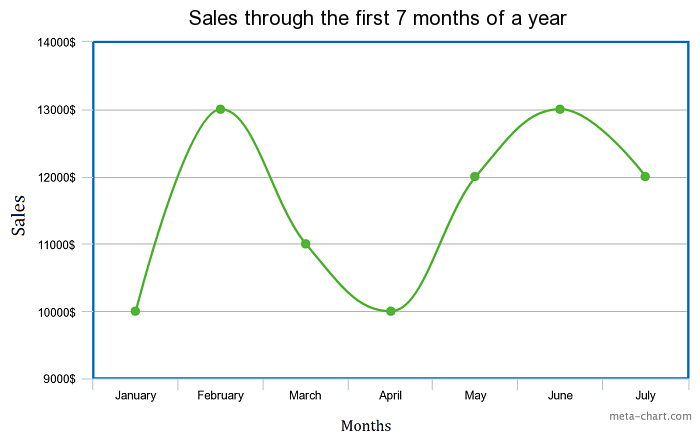9. Box and Whisker Chart

A box and whisker chart is a statistical graph for displaying sets of numerical data through their quartiles. It displays a frequency distribution of the data.

The box and whisker chart helps you to display the spread and skewness for a given set of data using the five number summary principle: minimum, maximum, median, lower and upper quartiles. The ‘five-number summary’ principle allows providing a statistical summary for a particular set of numbers. It shows you the range (minimum and maximum numbers), the spread (upper and lower quartiles), and the center (median) for the set of data numbers.

A very simple figure of a box and whisker plot you can see below: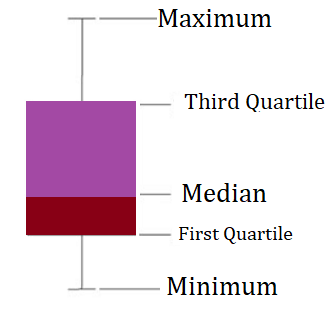Box and Whisker Chart Uses:

• When you want to observe the upper, lower quartiles, mean, median, deviations, etc. for a large set of data.
• When you want to see a quick view of the dataset distribution.
• When you have multiple data sets that come from independent sources and relate to each other in some way.
• When you need to compare data from different categories.

Example:

The table and box-and-whisker plots below shows test scores for Maths and Literature for the same class.

 Maths 35 77 92 43 55 66 73 70 Literature 35 43 40 43 50 60 70 92Box and Whisker charts have applications in many scientific areas and types of analysis such as statistical analysis, test results analysis, marketing analysis, data analysis, and etc.

10. Bubble Chart

Bubble charts are super useful types of graphs for making a comparison of the relationships between data in 3 numeric-data dimensions: the Y-axis data, the X-axis data, and data depicting the bubble size.

Bubble charts are very similar to XY Scatter plots but the bubble chart adds more functionality – a third dimension of data that can be extremely valuable.

Both axes (X and Y) of a bubble chart are numeric.

Bubble Chart Uses:

• When you have to display three or four dimensions of data.
• When you want to compare and display the relationships between categorized circles, by the use of proportions.

Example:

The bubble chart below shows the relationship between Cost (X-Axis), Profit (Y-Axis), and Probability of Success (%) (Bubble Size).11. Pictographs

The pictograph or a pictogram is one of the more visually appealing types of graphs and charts that display numerical information with the use of icons or picture symbols to represent data sets.

They are very easy to read statistical way of data visualization. A pictogram shows the frequency of data as images or symbols. Each image/symbol may represent one or more units of a given dataset.

Pictograph Uses:

• When your audience prefers and understands better displays that include icons and illustrations. Fun can promote learning.
• It’s habitual for infographics to use of a pictogram.
• When you want to compare two points in an emotionally powerful way.

Example:

The following pictographic represents the number of computers sold by a business company for the period from January to March.The pictographic example above shows that in January are sold 20 computers (4×5 = 20), in February are sold 30 computers (6×5 = 30) and in March are sold 15 computers.

12. Dot Plot

Dot plot or dot graph is just one of the many types of graphs and charts to organize statistical data. It uses dots to represent data. A Dot Plot is used for relatively small sets of data and the values fall into a number of discrete categories.

If a value appears more than one time, the dots are ordered one above the other. That way the column height of dots shows the frequency for that value.

Dot Plot Uses:

• To plot frequency counts when you have a small number of categories.
• Dot plots are very useful when the variable is quantitative or categorical.
• Dot graphs are also used for univariate data (data with only one variable that you can measure).

Example:

Suppose you have a class of 26 students. They are asked to tell their favorite color. The dot plot below represents their choices:It is obvious that blue is the most preferred color by the students in this class.

13. Radar Chart

A radar chart is one of the most modern types of graphs and charts – ideal for multiple comparisons. Radar charts use a circular display with several different quantitative axes looking like spokes on a wheel. Each axis shows a quantity for a different categorical value.

Radar charts are also known as spider charts, web charts, star plots, irregular polygons, polar charts, cobweb charts or Kiviat diagram.

Radar Chart has many applications nowadays in statistics, maths, business, sports analysis, data intelligence, and etc.

Radar Chart Uses:

• When you want to observe which variables have similar values or whether there are any outliers amongst each variable.
• To represent multiple comparisons.
• When you want to see which variables are scoring low or high within a dataset. This makes radar chart ideal for displaying performance.

Example:

For example, we can compare employee’s performance with the scale of 1-8 on subjects such as Punctuality, Problem-solving, Meeting Deadlines, Marketing Knowledge, Communications. A point that is closer to the center on an axis shows a lower value and a worse performance.

 Label Punctuality Problem-solving Meeting Deadlines Marketing Knowledge Communications Jane 6 5 8 7 8 Samanta 7 5 5 4 8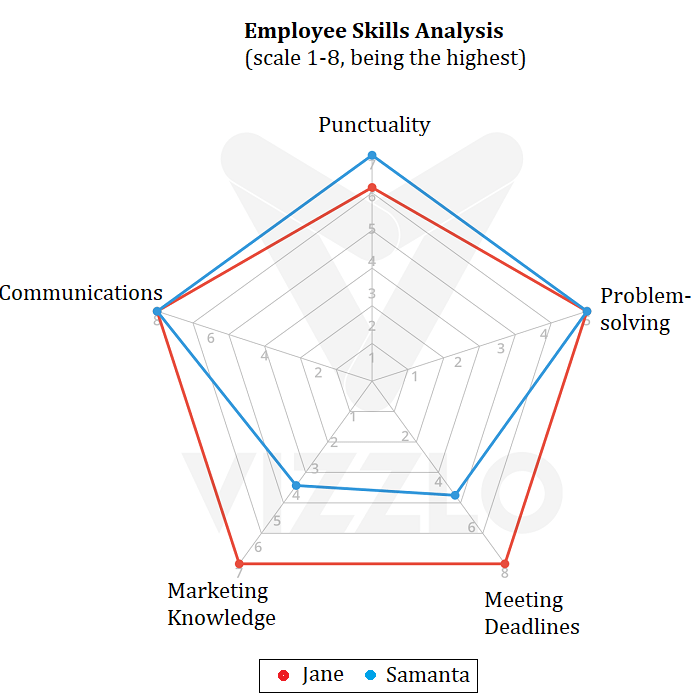It is obvious that Jane has a better performance than Samanta.

14. Pyramid Graph

When it comes to easy to understand and good looking types of graphs and charts, pyramid graph has a top place.

A pyramid graph is a chart in a pyramid shape or triangle shape. These types of charts are best for data that is organized in some kind of hierarchy. The levels show a progressive order.

Pyramid Graph Uses:

• When you want to indicate a hierarchy level among the topics or other types of data.
• Pyramid graph is often used to represent progressive orders such as: “older to newer”, “more important to least important”, “specific to least specific”‘ and etc.
• When you have a proportional or interconnected relationship between data sets.

Example:

A classic pyramid graph example is the healthy food pyramid that shows fats, oils, and sugar (at the top) should be eaten less than many other foods such as vegetables and fruits (at the bottom of the pyramid).Conclusion:

You might know that choosing the right type of chart is some kind of tricky business.

Practically, the choice depends on 2 major things: on the kind of analysis you are you want to perform and on the type of data you have.

Commonly, when we aim to facilitate a comparison, we use a bar chart or radar chart. When we want to show trends over time, we use a line chart or an area chart and etc.

Anyway, you have a wide choice of types of graphs and charts. Used in the right way, they are a powerful weapon to help you make your reports and presentations both professional and clear.

What are your favorite types of graphs and charts? Share your thoughts on the field below.